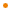# JavaFX: Bringing Rich Experiences To All the Screens Of Your Life

### Overview

The abstract class defines the single interpolate() method, which is used to calculate interpolated values. Various built-in implementations of this class are offered. Applications may choose to implement their own Interpolator to get custom interpolation behavior.

Profile: common

### Script Variable Summary

accessnametypedescription
publicDISCRETEInterpolatorBuilt-in interpolator that provides discrete time interpolation.Built-in interpolator that provides discrete time interpolation. The return value of `interpolate()` is `endValue` only when the input `faction` is 1.0, and `startValue` otherwise.

Profile: common

publicEASEBOTHInterpolatorBuilt-in interpolator instance that provides ease in/out behavior.Built-in interpolator instance that provides ease in/out behavior. It uses default values of 0.2 and 0.2 for the acceleration and deceleration factors, respectively.

Profile: common

publicEASEINInterpolatorBuilt-in interpolator instance that provides ease in behavior.Built-in interpolator instance that provides ease in behavior. It uses value of 0.2 for the acceleration factor.

Profile: common

publicEASEOUTInterpolatorBuilt-in interpolator instance that provides ease out behavior.Built-in interpolator instance that provides ease out behavior. It uses value of 0.2 for the deceleration factor.

Profile: common

publicLINEARInterpolatorBuilt-in interpolator that provides linear time interpolation.Built-in interpolator that provides linear time interpolation. The return value of `interpolate()` is `startValue` + (`endValue` - `startValue`) * `faction`.

Profile: common

### Script Function Summary

public SPLINE(x1: Number, y1: Number, x2: Number, y2: Number) : Interpolator

Built-in interpolator instance that is shaped using the spline control points defined by (`x1`, `y1`) and (`x2`, `y2`).Built-in interpolator instance that is shaped using the spline control points defined by (`x1`, `y1`) and (`x2`, `y2`). The anchor points of the spline are implicitly defined as (`0.0`, `0.0`) and (`1.0`, `1.0`).

Parameters
x1
x coordinate of the first control point
y1
y coordinate of the first control point
x2
x coordinate of the second control point
y2
y coordinate of the second control point
Returns
Interpolator
A spline interpolator

Profile: common

### Function Summary

public abstract interpolate(startValue: java.lang.Object, endValue: java.lang.Object, fraction: Number) : java.lang.Object

This function takes `startValue` and `endValue` along with `faction` between 0.0 and 1.0 and returns another value, between `startValue` and endValue.This function takes `startValue` and `endValue` along with `faction` between 0.0 and 1.0 and returns another value, between `startValue` and endValue. The purpose of the function is to define how time (represented as a (0.0 - 1.0) fraction of the duration of an animation) is altered to derive different value calculations during an animation.

Parameters
startValue
start value
endValue
end value
fraction
a value between 0.0 and 1.0
Returns
Object
interpolated value

Profile: common